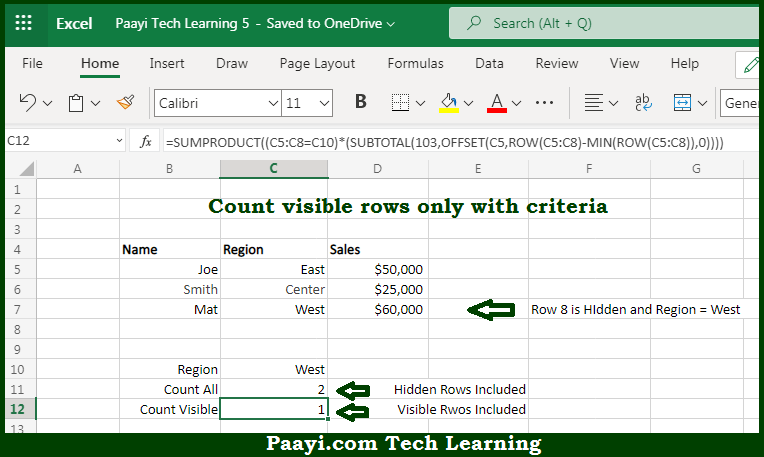# Learn How to COUNT Visible Rows Only With Criteria in Microsoft Excel

Written by | 0 Comments | 603 Views

In this article, you will learn how to COUNT various things in Microsoft Excel using a single or combination of functions and its purpose. You will also get to know how to COUNT Visible Rows Only With Criteria and see the generic formula.

COUNT Visible Rows Only With Criteria in Microsoft Excel

The main purpose of this formula is to count visible rows only with criteria. Here we will learn how to count visible rows only with given criteria in Microsoft Excel. That implies, with the help of a formula based on the SUBTOTAL, SUMPRODUCT, and OFFSET function you can able to count visible rows only with criteria. So, with the help of this formula, you can able to count visible rows only with given criteria in Microsoft Excel.

General Formula to COUNT Visible Rows Only With Criteria

=SUMPRODUCT((range=criteria)*(SUBTOTAL(103,OFFSET(range,rows,0,1))))

The Explanation for the COUNT Visible Rows Only With CriteriaSo we know that with the help of the given formula above you can able to count visible rows only with criteria. Here we will learn how to count visible rows only with given criteria in Microsoft Excel. As we know that the SUBTOTAL function can easily generate sums and counts for hidden and non-hidden rows. But, it isn't able to efficiently handle criteria like COUNTIF or SUMIF and needs some external help. One approach can be is to use the SUMPRODUCT function to apply both the SUBTOTAL function via OFFSET function and the criteria. So, with the help of this formula, you can able to count visible rows only with criteria.